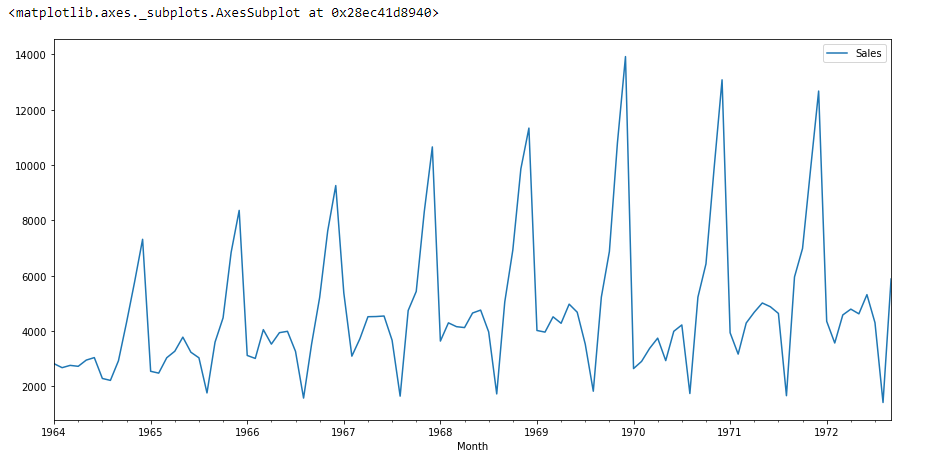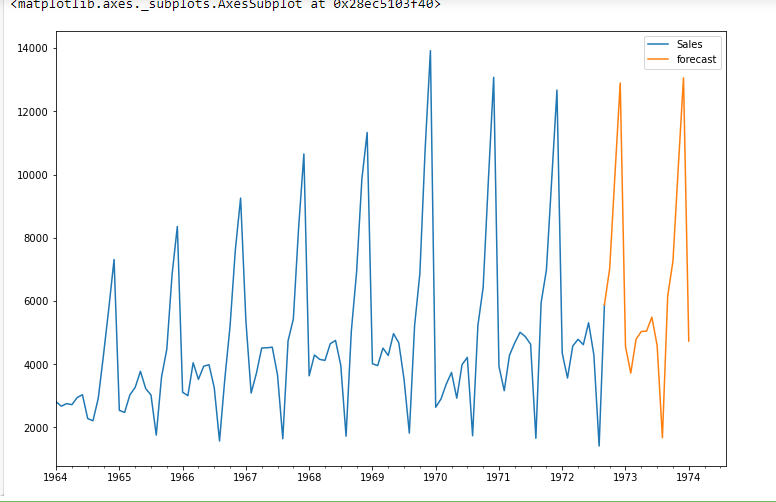Prabhat Pathak — Published On October 29, 2020 and Last Modified On August 25th, 2022

This article was published as a part of the Data Science Blogathon.

## Introduction

A popular and widely used statistical method for time series forecasting is the ARIMA model. Exponential smoothing and ARIMA models are the two most widely used approaches to time series forecasting and provide complementary approaches to the problem. While exponential smoothing models are based on a description of the trend and seasonality in the data, ARIMA models aim to describe the autocorrelations in the data.

Before we talk about the ARIMA model, let’s talk about the concept of stationarity and the technique of differencing time series.

## Stationarity

A stationary time series data is one whose properties do not depend on the time, That is why time series with trends, or with seasonality, are not stationary. the trend and seasonality will affect the value of the time series at different times, On the other hand for stationarity it does not matter when you observe it, it should look much the same at any point in time. In general, a stationary time series will have no predictable patterns in the long-term.

ARIMA is an acronym that stands for Auto-Regressive Integrated Moving Average. It is a class of model that captures a suite of different standard temporal structures in time series data.

In this tutorial, We will talk about how to develop an ARIMA model for time series forecasting in Python.

An ARIMA model is a class of statistical models for analyzing and forecasting time series data. It is really simplified in terms of using it, Yet this model is really powerful.

ARIMA stands for Auto-Regressive Integrated Moving Average.

The parameters of the ARIMA model are defined as follows:

p: The number of lag observations included in the model, also called the lag order.
d: The number of times that the raw observations are differenced, also called the degree of difference.
q: The size of the moving average window, also called the order of moving average.
A linear regression model is constructed including the specified number and type of terms, and the data is prepared by a degree of differencing in order to make it stationary, i.e. to remove trend and seasonal structures that negatively affect the regression model.

## STEPS

1. Visualize the Time Series Data
2. Identify if the date is stationary
3. Plot the Correlation and Auto Correlation Charts
4. Construct the ARIMA Model or Seasonal ARIMA based on the data

Let’s Start

```import numpy as np
import pandas as pd
import matplotlib.pyplot as plt
%matplotlib inline```

In this tutorial, I am using the below dataset.

```df=pd.read_csv('time_series_data.csv')

df.columns=["Month","Sales"]
df.describe()
df.set_index('Month',inplace=True)

from pylab import rcParams
rcParams['figure.figsize'] = 15, 7
df.plot()```if we see the above graph then we will able to find a trend that there is a time when sales are high and vice versa. That means we can see data is following seasonality. For ARIMA first thing we do is identify if the data is stationary or non – stationary. if data is non-stationary we will try to make them stationary then we will process further.

Let’s check that if the given dataset is stationary or not, For that we use adfuller.

`from statsmodels.tsa.stattools import adfuller`

I have imported the adfuller by running the above code.

`test_result=adfuller(df['Sales'])`

To identify the nature of data, we will be using the null hypothesis.

H0: The null hypothesis: It is a statement about the population that either is believed to be true or is used to put forth an argument unless it can be shown to be incorrect beyond a reasonable doubt.

H1: The alternative hypothesis: It is a claim about the population that is contradictory to H0 and what we conclude when we reject H0.

#Ho: It is non-stationary
#H1: It is stationary

We will be considering the null hypothesis that data is not stationary and the alternate hypothesis that data is stationary.

```def adfuller_test(sales):
labels = ['ADF Test Statistic','p-value','#Lags Used','Number of Observations']
for value,label in zip(result,labels):
print(label+' : '+str(value) )

if result <= 0.05:
print("strong evidence against the null hypothesis(Ho), reject the null hypothesis. Data is stationary")
else:
print("weak evidence against null hypothesis,indicating it is non-stationary ")

After running the above code we will get P-value,

```ADF Test Statistic : -1.8335930563276237
p-value : 0.3639157716602447
#Lags Used : 11
Number of Observations : 93```

Here P-value is 0.36 which is greater than 0.05, which means data is accepting the null hypothesis, which means data is non-stationary.

Let’s try to see the first difference and seasonal difference:

```df['Sales First Difference'] = df['Sales'] - df['Sales'].shift(1)
df['Seasonal First Difference']=df['Sales']-df['Sales'].shift(12)
```# Again testing if data is stationary
```ADF Test Statistic : -7.626619157213163
p-value : 2.060579696813685e-11
#Lags Used : 0
Number of Observations : 92```

Here P-value is 2.06, which means we will be rejecting the null hypothesis. So data is stationary.

`df['Seasonal First Difference'].plot()`

I am going to create auto-correlation :

```from pandas.plotting import autocorrelation_plot
autocorrelation_plot(df['Sales'])
plt.show()```
```from statsmodels.graphics.tsaplots import plot_acf,plot_pacf
import statsmodels.api as sm
fig = plt.figure(figsize=(12,8))
fig = sm.graphics.tsa.plot_acf(df['Seasonal First Difference'].dropna(),lags=40,ax=ax1)
fig = sm.graphics.tsa.plot_pacf(df['Seasonal First Difference'].dropna(),lags=40,ax=ax2)```
```# For non-seasonal data
#p=1, d=1, q=0 or 1

from statsmodels.tsa.arima_model import ARIMA
model=ARIMA(df['Sales'],order=(1,1,1))
model_fit=model.fit()
model_fit.summary()```
Dep. Variable: No. Observations: D.Sales 104 ARIMA(1, 1, 1) -951.126 css-mle 2227.262 Wed, 28 Oct 2020 1910.251 11:49:08 1920.829 02-01-1964 1914.536 – 09-01-1972

coef std err z P>|z| [0.025 0.975] 22.7845 12.405 1.837 0.066 -1.529 47.098 0.4343 0.089 4.866 0.000 0.259 0.609 -1.0000 0.026 -38.503 0.000 -1.051 -0.949

Real Imaginary Modulus Frequency 2.3023 +0.0000j 2.3023 0.0000 1.0000 +0.0000j 1.0000 0.0000

```df['forecast']=model_fit.predict(start=90,end=103,dynamic=True)
df[['Sales','forecast']].plot(figsize=(12,8))```
```import statsmodels.api as sm
model=sm.tsa.statespace.SARIMAX(df['Sales'],order=(1, 1, 1),seasonal_order=(1,1,1,12))
results=model.fit()
df['forecast']=results.predict(start=90,end=103,dynamic=True)
df[['Sales','forecast']].plot(figsize=(12,8))```
```from pandas.tseries.offsets import DateOffset
future_dates=[df.index[-1]+ DateOffset(months=x)for x in range(0,24)]
future_datest_df=pd.DataFrame(index=future_dates[1:],columns=df.columns)

future_datest_df.tail()

future_df=pd.concat([df,future_datest_df])

future_df['forecast'] = results.predict(start = 104, end = 120, dynamic= True)
future_df[['Sales', 'forecast']].plot(figsize=(12, 8))```## Conclusion

Time Series forecasting is really useful when we have to take future decisions or we have to do analysis, we can quickly do that using ARIMA, there are lots of other Models from we can do the time series forecasting but ARIMA is really easy to understand.

HAPPY CODING.

Prabhat Pathak (Linkedin profile) is a Senior Analyst.## 4 thoughts on "How to Create an ARIMA Model for Time Series Forecasting in Python"###### Gaurav Sinha says:October 30, 2020 at 1:41 pm
Very nice and detailed article. Thanks for sharing! Reply###### Gaurang says:October 31, 2020 at 7:55 pm